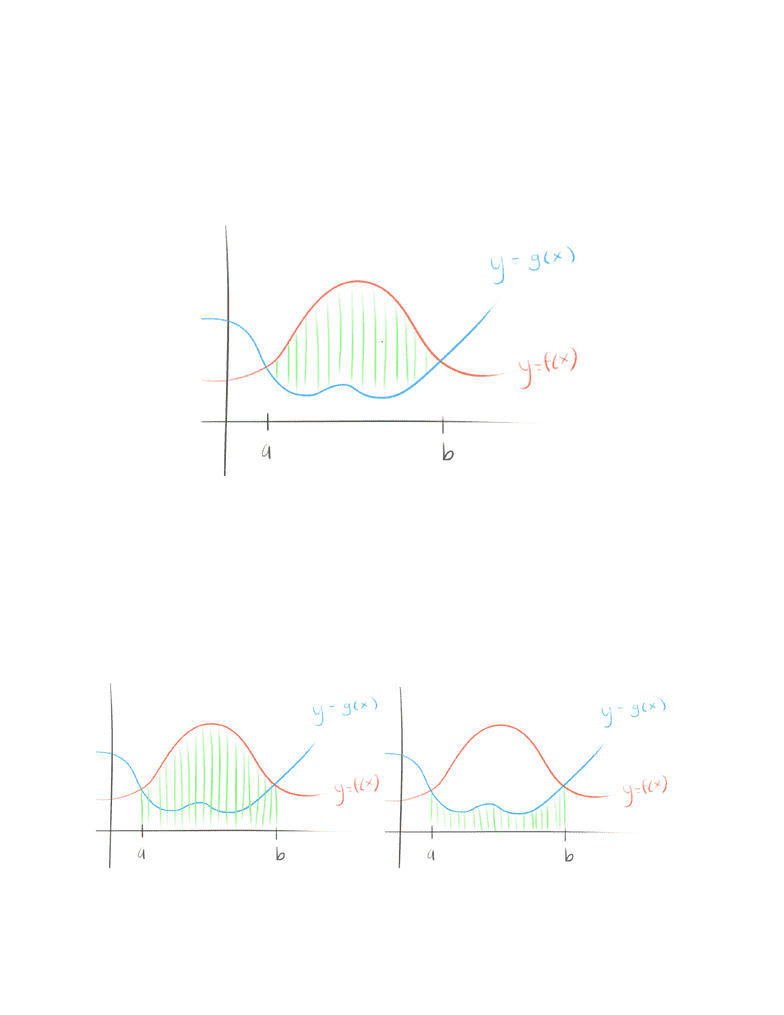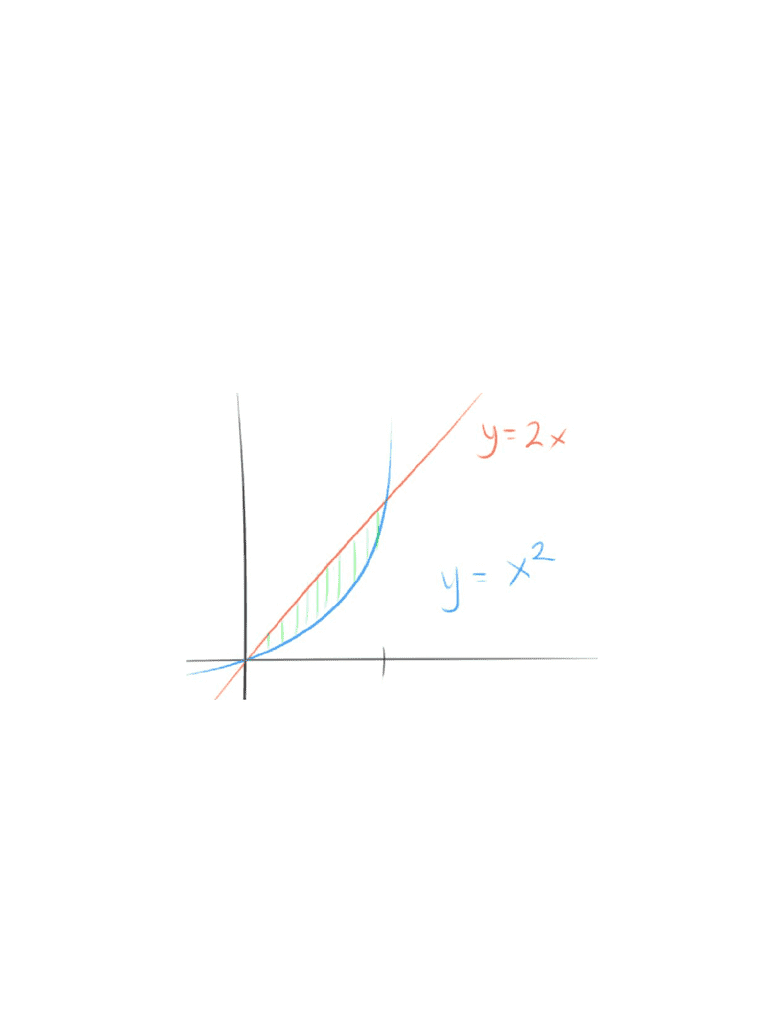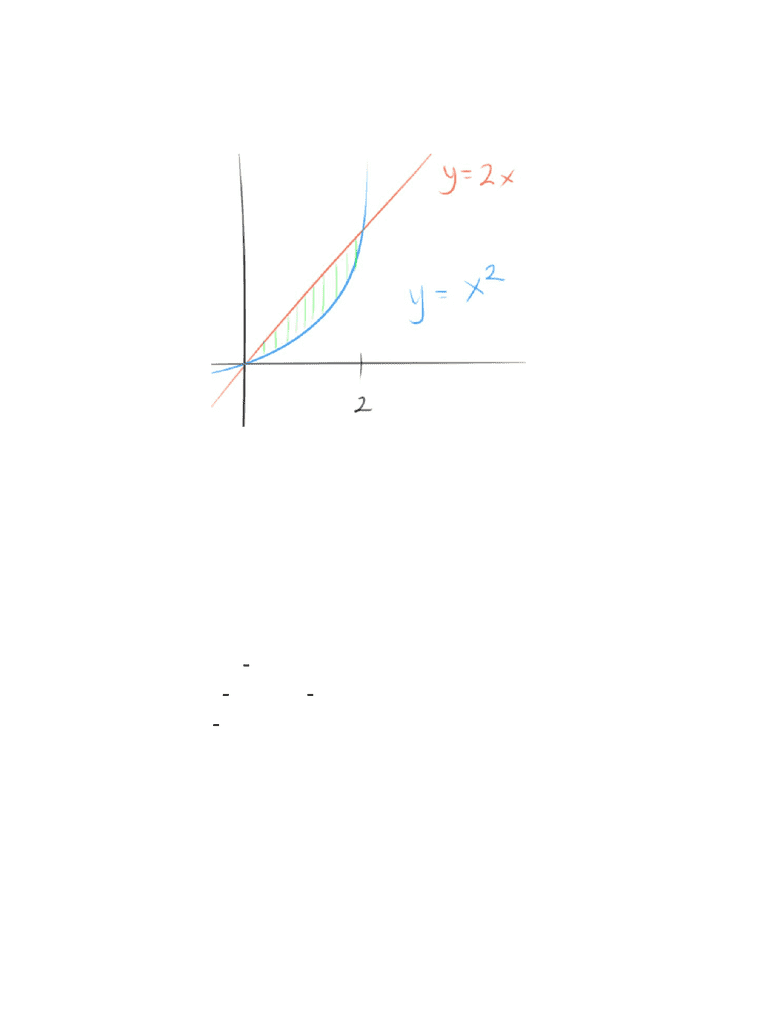Study Guides (390,000)
US (220,000)
UC-Irvine (3,000)
MATH (600)
MATH 2B (100)
All (60)
Midterm

# MATH 2B Midterm: MATH2B, Chapter 6 term test 1Premium

Department
Mathematics
Course Code
MATH 2B
Professor
All
Study Guide
Midterm

This preview shows pages 1-3. to view the full 9 pages of the document.Find the Area of the Region
What we want to do is take the integral, which would give us the area under the curve
However, if we were to take the integral of or only, you wouldn’t quite get(x)g(x)f
the area highlighted in green that we want
What to do?
Take the area of (x) g(x)f
Area Between Curves
Section 6.1

Only pages 1-3 are available for preview. Some parts have been intentionally blurred.

Subscribers OnlyWhy? Because the area under the curve of subtracted from the area under(x)g
the curve of would give us the desired area.(x)f
What would this give us?
(x) g(x)dxA =
b
a
f
OP (x) BOT (x)dxA =
b
a
T
In other words, the area between the curves is the area of the top curve
minus the bottom curve
Example #1
Find the area between and 2x y= xy = 2
Firstly, we want to define the boundaries that we will take the area under
Find the intersection points of the two functions
Set the functions equal to each other to do so
x x2 = 2
x 2x0 = 2

Only pages 1-3 are available for preview. Some parts have been intentionally blurred.

Subscribers Onlyx(x 2)0 =
, 2x= 0x=
So we will take the integral from [0, 2]
x x dx
2
0
2 2
We know that we can compile the new integral like so because from the graph,
we can clearly see that is clearly on the top 2xy =
x x dxA =
2
0
2 2
x(− x)= 23
13
2 2 ) (0 0 )( 23
13 23
13
= 3
4
Example #2
Find the area between the curves 4, y 2 , x 1y= = x =